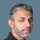cancel
Showing results for
Did you mean:Helper III

## Evaluation based on several conditions

Hi all,

I would like to ask you for help with DAX formula for this case:

I have the table with order number, operation number and code:

 ORDER OPERATION CODE 111 0100 01 111 0100 02 111 0200 01 111 0200 02 222 0110 01 222 0110 02 222 0210 01

Each operation have 01 code which means that operation started and 02 code when the operation was closed. And what I want to do is identify which order with specific operation is open or closed.

So I need to create new measure/column where will be defined following rules:

- if operation number containts "02" code than it is closed

- if operation number doesn´t containts code "02" than it is open

Here is desired output:

 ORDER OPERATION CODE STATUS 111 0100 01 closed 111 0100 02 closed 111 0200 01 closed 111 0200 02 closed 222 0110 01 closed 222 0110 02 closed 222 0210 01 open

All orders and operations contains "02" code therefore are closed only order 222 with operation 0210 doesn´t have "02" code so therefore is open...

Any idea how to define the formula?

P.

1 ACCEPTED SOLUTIONSuper User

@Peter_2020

``````Status =
VAR __OR = Table1[ORDER]
VAR __OP = Table1[OPERATION]
VAR __CODE =
CALCULATETABLE(
VALUES(Table1[CODE]),
Table1[ORDER] = __OR,
Table1[OPERATION] = __OP,
REMOVEFILTERS(Table1)
)
VAR __RESULT =
IF(
"02" IN __CODE,
"CLOSED",
"OPEN"
)
RETURN
__RESULT
``````If you like a measure, this should work:

``````M1 =
VAR __CODE =
VALUES(Table1[CODE])
VAR __RESULT =
IF(
"02" IN __CODE,
"CLOSED",
"OPEN"
)
RETURN
IF(
HASONEVALUE(Table1[OPERATION]),
__RESULT
)``````Did I answer your question? Mark my post as a solution! and hit thumbs up
2 REPLIES 2Helper III

@Fowmy Thank you very much. It works!Super User

@Peter_2020

``````Status =
VAR __OR = Table1[ORDER]
VAR __OP = Table1[OPERATION]
VAR __CODE =
CALCULATETABLE(
VALUES(Table1[CODE]),
Table1[ORDER] = __OR,
Table1[OPERATION] = __OP,
REMOVEFILTERS(Table1)
)
VAR __RESULT =
IF(
"02" IN __CODE,
"CLOSED",
"OPEN"
)
RETURN
__RESULT
``````If you like a measure, this should work:

``````M1 =
VAR __CODE =
VALUES(Table1[CODE])
VAR __RESULT =
IF(
"02" IN __CODE,
"CLOSED",
"OPEN"
)
RETURN
IF(
HASONEVALUE(Table1[OPERATION]),
__RESULT
)``````Did I answer your question? Mark my post as a solution! and hit thumbs upAnnouncements#### Exclusive opportunity for Women!

Join us for a free, hands-on Microsoft workshop led by women trainers for women where you will learn how to build a Dashboard in a Day!#### Power Platform Conference-Power BI and Fabric Sessions

Join us Oct 1 - 6 in Las Vegas for the Microsoft Power Platform Conference.Top Solution Authors
Top Kudoed Authors
Users online (1,723)